2393字，阅读需时8分钟① 理解牛顿迭代法；

②掌握使用牛顿迭代法计算任意正实数近似平方根的算法。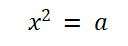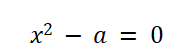（1）方程V变量x赋初值x0，作为方程的近似根；

① 计算方程V的下一个近似根x1；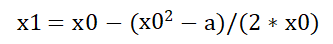② 计算x0和x1差的绝对值differ；

③ 若differ小于指定的数值，则认为x1为方程V的近似根，执行第④个步骤，否则继续执行迭代；

④ 返回x1，迭代结束

Python代码

```import math
# 计算实数平方根的方程
def f1(x,a):
return x*x-a
# 计算实数平方根方程的导函数
def f2(x):
return 2*x

# 计算实数的平方根
def sqrt(a):
# x0为方程的初始值，作为方程的初始近似根
x0 = a/2
# 计算方程的下一个近似根x1
x1 = x0 - f1(x0,a)/f2(x0);
# 计算两个近似根x0和x1差的绝对值
differ = math.fabs(x1-x0)
# 循环计算方程的近似根，直至两个近似根差的绝对值小于1e-5
while( differ >= 1e-5 ):
# x0被赋值为x1
x0 = x1
# 计算方程的下一个近似根x1
x1 = x0 - f1(x0,a)/f2(x0);
# 计算两个近似根x0和x1的绝对差
differ = math.fabs(x1-x0)
return x1

# 程序入口
if __name__ == '__main__':

a = input("请输入一个正实数:\n")
print("%.5f" % sqrt(float(a)))```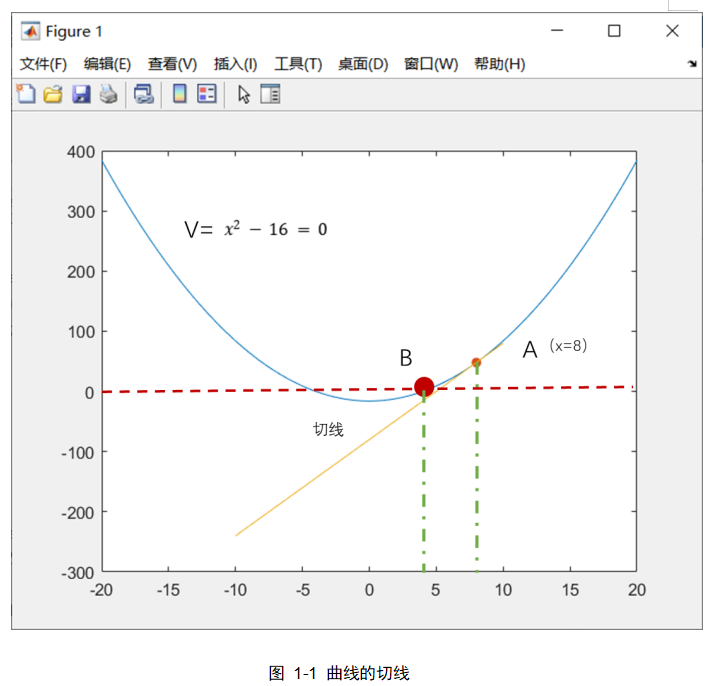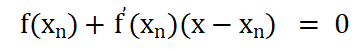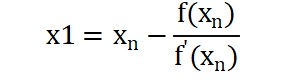x1是过A点切线方程与X轴交点的横坐标，过点（x1，f(x1)）继续做方程V的切线，由过点（x1，f(x1)）的切线方程计算得到x2。依次类推，直至A点移动到B点或x1与x2差的绝对值小于指定的一个非常小的数，整个迭代结束。

```% 在区间[-1,1]内创建100个x坐标数据点
x = linspace(-20,20,100);
% 计算f(x)=x^2函数的y坐标
y = x.^2-16;
% 绘制曲线
plot(x,y)
hold on
% 绘制曲线点（x=8）的切线
draw_line(8,8*8-16)

% 定义绘制切线函数
function draw_line(x,y)
% 绘制数据点
scatter(x,y,'filled')
% 计算函数y=x^2曲线（x,y）点的切线斜率
k = 2 * x
x1 = linspace(-10,10,100)
% 通过切线方程计算y数据
y1 = k*(x1-x)+y
% 绘制切线
plot(x1,y1)
End```